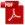### 10.2.1. Membrane Action of Plates under Uniform Pressure

Reference: Abbott, Richard. Analysis and Design of Composite and Metallic Flight Vehicle Structures 3 Edition, 2019.

This section is taken substantially from  (AFFDL-TR-69-42, 1986) which has a good succinct summary of relevant analysis methods.

A membrane is a plate that has no bending rigidity, all stresses are in the plane of the surface and are usually tension.

This section uses the following nomenclature:

Where:

10.2.1.1 Circular Membranes

The maximum deflection of a circular membrane with a clamped edge is given by:

The deflection of the membrane at a distance, r, from the center of the plate is:

The stress at the center of the membrane is:

The stress at the edge of the membrane is:

This method is available in a spreadsheet:

10.2.1.2 Rectangular Membranes

For a long membrane, the behavior will be such that the plate will behave the same whether the short edges are restrained.

A membrane is assumed to be ‘long’ if a / b > 5.

A long plate is considered to be a membrane if P/E · (b/t)⁴ is greater than 100.

The maximum defection at the center of the membrane is given by:

The stress at the center of the membrane is:

For a short membrane, a / b < 5

Where n1 thru n7 are coefficients determined using the following figure:

The deflection at the center of the membrane is given by:

The stresses at the center of the membrane are given by:

The stresses at the center of the short side are given by:

The stresses at the center of the long side are given by:

The maximum stresses occur at the center of the long side of the plate.

This method is available as a spreadsheet here:

### 10.2.1. Membrane Action of Plates under Uniform Pressure

Reference: Abbott, Richard. Analysis and Design of Composite and Metallic Flight Vehicle Structures 3 Edition, 2019.

This section is taken substantially from  (AFFDL-TR-69-42, 1986) which has a good succinct summary of relevant analysis methods.

A membrane is a plate that has no bending rigidity, all stresses are in the plane of the surface and are usually tension.

This section uses the following nomenclature:

Where:

10.2.1.1 Circular Membranes

The maximum deflection of a circular membrane with a clamped edge is given by:

The deflection of the membrane at a distance, r, from the center of the plate is:

The stress at the center of the membrane is:

The stress at the edge of the membrane is:

This method is available in a spreadsheet:

10.2.1.2 Rectangular Membranes

For a long membrane, the behavior will be such that the plate will behave the same whether the short edges are restrained.

A membrane is assumed to be ‘long’ if a / b > 5.

A long plate is considered to be a membrane if P/E · (b/t)⁴ is greater than 100.

The maximum defection at the center of the membrane is given by:

The stress at the center of the membrane is:

For a short membrane, a / b < 5

Where n1 thru n7 are coefficients determined using the following figure: